x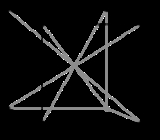Coordination numberEncyclopedia
In chemistry
Chemistry
Chemistry is the science of matter, especially its chemical reactions, but also its composition, structure and properties. Chemistry is concerned with atoms and their interactions with other atoms, and particularly with the properties of chemical bonds....

and crystallography
Crystallography
Crystallography is the experimental science of the arrangement of atoms in solids. The word "crystallography" derives from the Greek words crystallon = cold drop / frozen drop, with its meaning extending to all solids with some degree of transparency, and grapho = write.Before the development of...

, the coordination number of a central atom in a molecule
Molecule
A molecule is an electrically neutral group of at least two atoms held together by covalent chemical bonds. Molecules are distinguished from ions by their electrical charge...

or crystal
Crystal
A crystal or crystalline solid is a solid material whose constituent atoms, molecules, or ions are arranged in an orderly repeating pattern extending in all three spatial dimensions. The scientific study of crystals and crystal formation is known as crystallography...

is the number of its nearest neighbours. This number is determined somewhat differently for molecules and for crystals.

In chemistry, the emphasis is on bonding structure in molecules or ions and the coordination number of an atom is determined by simply counting the other atoms to which it is bonded (by either single or multiple bonds). For example, [Cr(NH3)2Cl2Br2]1- has Cr3+ as its central cation, which has a coordination number of 6.

However the solid-state structures of crystals often have less clearly defined bonds
Chemical bond
A chemical bond is an attraction between atoms that allows the formation of chemical substances that contain two or more atoms. The bond is caused by the electromagnetic force attraction between opposite charges, either between electrons and nuclei, or as the result of a dipole attraction...

, so a simpler model is used, in which the atoms are represented by touching spheres. In this model the coordination number of an atom is the number of other atoms that it touches. For an atom in the interior of a crystal lattice, the number of atoms touching the given atom is the bulk coordination number; for an atom at a surface of a crystal, this is the surface coordination number.

## Chemistry usage

In chemistry, coordination number (c.n.), defined originally in 1893 by Alfred Werner
Alfred Werner
Alfred Werner was a Swiss chemist who was a student at ETH Zurich and a professor at the University of Zurich. He won the Nobel Prize in Chemistry in 1913 for proposing the octahedral configuration of transition metal complexes. Werner developed the basis for modern coordination chemistry...

, is the total number of neighbours of a central atom in a molecule or ion. Although a carbon atom has four chemical bonds in most stable molecules, the coordination number of each carbon
Carbon
Carbon is the chemical element with symbol C and atomic number 6. As a member of group 14 on the periodic table, it is nonmetallic and tetravalent—making four electrons available to form covalent chemical bonds...

is four in methane
Methane
Methane is a chemical compound with the chemical formula . It is the simplest alkane, the principal component of natural gas, and probably the most abundant organic compound on earth. The relative abundance of methane makes it an attractive fuel...

(CH4), three in ethylene
Ethylene
Ethylene is a gaseous organic compound with the formula . It is the simplest alkene . Because it contains a carbon-carbon double bond, ethylene is classified as an unsaturated hydrocarbon. Ethylene is widely used in industry and is also a plant hormone...

(H2C=CH2, each C is bonded to 2H + 1C = 3 atoms), and two in acetylene
Acetylene
Acetylene is the chemical compound with the formula C2H2. It is a hydrocarbon and the simplest alkyne. This colorless gas is widely used as a fuel and a chemical building block. It is unstable in pure form and thus is usually handled as a solution.As an alkyne, acetylene is unsaturated because...

. In effect we count the first bond (or sigma bond
Sigma bond
In chemistry, sigma bonds are the strongest type of covalent chemical bond. They are formed by head-on overlapping between atomic orbitals. Sigma bonding is most clearly defined for diatomic molecules using the language and tools of symmetry groups. In this formal approach, a σ-bond is...

) to each neighbouring atom, but not the other bonds (pi bond
Pi bond
In chemistry, pi bonds are covalent chemical bonds where two lobes of one involved atomic orbital overlap two lobes of the other involved atomic orbital...

s).

In inorganic chemistry
Inorganic chemistry
Inorganic chemistry is the branch of chemistry concerned with the properties and behavior of inorganic compounds. This field covers all chemical compounds except the myriad organic compounds , which are the subjects of organic chemistry...

also, only the first or sigma bond between each ligand
Ligand
In coordination chemistry, a ligand is an ion or molecule that binds to a central metal atom to form a coordination complex. The bonding between metal and ligand generally involves formal donation of one or more of the ligand's electron pairs. The nature of metal-ligand bonding can range from...

and the central atom counts, but not any pi bonds to the same ligands. In tungsten hexacarbonyl
Tungsten hexacarbonyl
Tungsten hexacarbonyl is the chemical compound with the formula W6. This complex gave rise to the first example of a dihydrogen complex....

, W(CO)6, the coordination number of tungsten (W) is counted as six although pi as well as sigma bonding is important in such metal carbonyl
Metal carbonyl
Metal carbonyls are coordination complexes of transition metals with carbon monoxide ligands. These complexes may be homoleptic, that is containing only CO ligands, such as nickel carbonyl , but more commonly metal carbonyls contain a mix of ligands, such as Re3Cl...

s.

Examples of high coordination number complexes are the ions formed by uranium
Uranium
Uranium is a silvery-white metallic chemical element in the actinide series of the periodic table, with atomic number 92. It is assigned the chemical symbol U. A uranium atom has 92 protons and 92 electrons, of which 6 are valence electrons...

and thorium
Thorium
Thorium is a natural radioactive chemical element with the symbol Th and atomic number 90. It was discovered in 1828 and named after Thor, the Norse god of thunder....

with bidentate nitrate
Nitrate
The nitrate ion is a polyatomic ion with the molecular formula NO and a molecular mass of 62.0049 g/mol. It is the conjugate base of nitric acid, consisting of one central nitrogen atom surrounded by three identically-bonded oxygen atoms in a trigonal planar arrangement. The nitrate ion carries a...

ion ligands, U(NO3)62− and Th(NO3)62−. Here each nitrate ligand is bound to the metal by two oxygen atoms, so that the total coordination number of the U or Th atom is 12.

When the surrounding ligands get smaller even higher coordination numbers may be possible. One computational chemistry
Computational chemistry
Computational chemistry is a branch of chemistry that uses principles of computer science to assist in solving chemical problems. It uses the results of theoretical chemistry, incorporated into efficient computer programs, to calculate the structures and properties of molecules and solids...

study predicted a particularly stable PbHe152+ ion composed of a central lead
Lead is a main-group element in the carbon group with the symbol Pb and atomic number 82. Lead is a soft, malleable poor metal. It is also counted as one of the heavy metals. Metallic lead has a bluish-white color after being freshly cut, but it soon tarnishes to a dull grayish color when exposed...

ion coordinated with no fewer than 15 helium atoms.

## Crystallography usage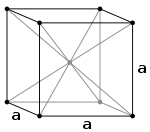In materials science
Materials science
Materials science is an interdisciplinary field applying the properties of matter to various areas of science and engineering. This scientific field investigates the relationship between the structure of materials at atomic or molecular scales and their macroscopic properties. It incorporates...

, the bulk coordination number of a given atom in the interior of a crystal lattice is the number of atoms touching the given atom. Iron at 20oC has a body-centered cubic (BCC) crystal
Cubic crystal system
In crystallography, the cubic crystal system is a crystal system where the unit cell is in the shape of a cube. This is one of the most common and simplest shapes found in crystals and minerals....

in which each interior iron atom occupies the centre of a cube formed by eight neighbouring iron atoms. The bulk coordination number for this structure is therefore 8.

The highest bulk coordination number is 12, found in both hexagonal close-packed (HCP) and cubic close-packed (CCP) (also known as face-centered cubic
Cubic crystal system
In crystallography, the cubic crystal system is a crystal system where the unit cell is in the shape of a cube. This is one of the most common and simplest shapes found in crystals and minerals....

or FCC) structures. This value of 12 corresponds to the theoretical limit of the kissing number problem
Kissing number problem
In geometry, a kissing number is defined as the number of non-overlapping unit spheres that touch another given unit sphere. For a lattice packing the kissing number is the same for every sphere, but for an arbitrary sphere packing the kissing number may vary from one sphere to another...

when all spheres are identical.The two most common allotropes of carbon have different coordination numbers. In diamond
Diamond
In mineralogy, diamond is an allotrope of carbon, where the carbon atoms are arranged in a variation of the face-centered cubic crystal structure called a diamond lattice. Diamond is less stable than graphite, but the conversion rate from diamond to graphite is negligible at ambient conditions...

, each carbon atom is at the centre of a tetrahedron
Tetrahedron
In geometry, a tetrahedron is a polyhedron composed of four triangular faces, three of which meet at each vertex. A regular tetrahedron is one in which the four triangles are regular, or "equilateral", and is one of the Platonic solids...

formed by four other carbon atoms, so the coordination number is four, as for methane. Graphite
Graphite
The mineral graphite is one of the allotropes of carbon. It was named by Abraham Gottlob Werner in 1789 from the Ancient Greek γράφω , "to draw/write", for its use in pencils, where it is commonly called lead . Unlike diamond , graphite is an electrical conductor, a semimetal...

is made of two-dimensional layers in which each carbon is covalently bonded to three other carbons. Atoms in other layers are much further away and are not nearest neighbours, so the coordination number of a carbon atom in graphite is 3 as in ethylene.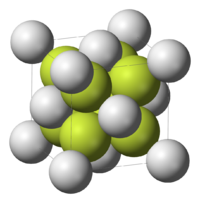Simple ionic
Ionic crystal
An ionic crystal is a crystal consisting of ions bound together by their electrostatic attraction. Examples of such crystals are the alkali halides, including potassium fluoride, potassium chloride, potassium bromide, potassium iodide, sodium fluoride, and other combinations of sodium, caesium,...

structures are described by two coordination numbers, one for each type of ion. Calcium fluoride
Calcium fluoride
Calcium fluoride is the inorganic compound with the formula CaF2. This ionic compound of calcium and fluorine occurs naturally as the mineral fluorite . It is the source of most of the world's fluorine. This insoluble solid adopts a cubic structure wherein calcium is coordinated to eight fluoride...

(CaF2) is an (8, 4) structure, meaning that each cation Ca2+ is surrounded by eight F anion neighbors, and each anion F by four Ca2+. For sodium chloride
Sodium chloride
Sodium chloride, also known as salt, common salt, table salt or halite, is an inorganic compound with the formula NaCl. Sodium chloride is the salt most responsible for the salinity of the ocean and of the extracellular fluid of many multicellular organisms...

(NaCl), the numbers of cations and anions are equal, and both coordination numbers are six so that the structure is (6, 6).

For an atom at a surface of a crystal, the surface coordination number is always less than the bulk coordination number. The surface coordination number is dependent on the Miller indices
Miller index
Miller indices form a notation system in crystallography for planes and directions in crystal lattices.In particular, a family of lattice planes is determined by three integers h, k, and ℓ, the Miller indices. They are written , and each index denotes a plane orthogonal to a direction in the...

of the surface. In a body-centered cubic (BCC) crystal
Cubic crystal system
In crystallography, the cubic crystal system is a crystal system where the unit cell is in the shape of a cube. This is one of the most common and simplest shapes found in crystals and minerals....

, the bulk coordination number is 8, whereas, for the (100) surface, the surface coordination number is 4.

## Usage in quasicrystal, liquid and other disordered systems

The coordination number of systems with disorderness cannot be precisely defined.

The first coordination number can be defined using the radial distribution function
In statistical mechanics, a radial distribution function , g, describes how the atomic density varies as a function of the distance from one particular atom....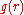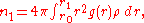where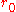is the rightmost position starting from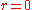whereonis approximately zero,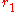is the first minimum. Therefore, it is the area under the first peak of.
The second coordinate number is defined similarly: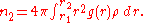Alternative definitions for the coordination number can be found in literature, but in essence the main idea is the same. One of those definition are as follows: Denote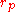as the position of the first peak,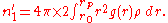The first coordination shell is the spherical shell with radius betweentoaround the central particle under investigation.